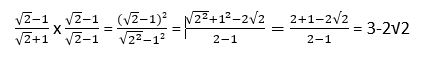# Checkerboard puzzler question and answer are both wrong

The question and answer both refer to removing a black and a red square, when the diagonally opposite squares are both either red, or black:

With that correction, the puzzler works, because you can’t cover the modified board with dominoes if each has to cover one black and one red square.

The Checkerboard | Car Talk

I’m not sure what you mean. The puzzler seems to say to remove two squares sharing a diagonal, say one at the top right, and one at the bottom left. So the two you remove would be the same color.

Are you referring to the comment that the two squares removed would be different colors? If so, which two squares do you think are removed?

Here’s what the puzzler says:

“So now, you have a checkerboard with 62 squares, because you just cut out two of them. The two are diagonally across from either other on the board. One black square and one red square.”

That is incorrect, the two squares “diagonally across from each other on the board” would have to be the same color, not “One black square and one red square.”

The answer is correct though. If two of the same color are removed, then two squares of the other color will be left, and will be impossible to cover. So there is no solution.

The answer says it the wrong way, though: “Because you removed one red and one black square, you are now unable to line up the dominoes to each cover one red and one black square.”

It should say “Because you removed two squares of the same color, you are now unable to line up the dominoes to each cover one red and one black square.”

Remove any square, if you remove a red one 2 diagonal reds,same for black except for corner squares. Shirley am I missing something?
.

Good catch. Presumably, they were using the Mutilated Chessboard Problem where diagonally opposite corners are removed. If you removed a red and a black corner (same side), it would be easy to cover the resulting board in 31 dominoes.

No doubt, the puzzle is stated a little incorrectly. It’s a well-known puzzle, and when I’ve come across it before, they have always removed two squares at each end of the same diagonal, so both were the same color. In those versions don’t think the dominos are required to cover a red/black combo, any two squares you want. But since covering a red/black combo with a domino is the only possibility, that is the proof why it can’t be done.

Here’s an interesting puzzle. Math theory usually says you have to have as many equations as you have unknowns to find a unique answer. But it is possible sometimes to find a unique answer even with just one equation and three unknowns. For example

a x b x c =30?
“x” means multiplication.

One equation, three unknowns, a, b, & c are all whole numbers. clue: very easy

1x5x6
2x3x5

Oops… you caught me on that one … lol … rigorously speaking I guess the answer I was thinking of (second in your list) isn’t “unique” b/c a=2, b=3, c=5 is just one solution. a=3, b=5, c=2 would work too, etc. .

Also,

1x2x15
1x3x10

1 Like

1 x 1 x 30 would work too … lol … there’s a sort of unwritten rule among math-types that answers should be restricted to avoid ambiguities. In this case it would usually be to prime numbers.

Likewise If you gave 1/sqrt(2) as an answer in a math class you might get it wrong. It should be expressed as sqrt(2)/2. Although both are the same number.

When I was in school, (admitedly that was long ago) you were supposed to reduce wherever possible, but you showed all work, so 1x1x30 would still be acceptable

An 11.11.23 puzzle in the Guardian Newspaper asked if one of the following should be marked “wrong” , student answers to the same test question. What do you think?

• (sqrt(2) -1)/(sqrt(2) + 1)

• 3 - 2*sqrt(2)

I think any math teacher is going to mark (√2-1)/(√2+1) wrong because it can be rationalized to 3-2√2.1 Like

+1. I seem to recall a convention to eliminate roots in the denominator when possible.

Good recollection. Square roots always need to be removed from the denominator of a fraction because the square root of a perfect square (1, 4, 9, 16, etc.) is a whole number which is easily removed. And, the square root of any non-perfect square is an irrational number. You can’t have an irrational number in the denominator because it has no definitive value.